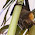## Monday, July 13, 2015

### Is there an easier proof? A less messy proof?

Consider the following statement:

BEGIN STATEMENT:

For all a,b,c, the equations

x + y + z = a

x2 +y2 + z2 = b

x3 + y3 + z3 = c

has a unique solution (up to perms of x,y,z).

END STATEMENT

One can also look at this with k equations, k variables, and powers 1,2,...,k.

The STATEMENT is true. One can use Newton's  identities (see here) to obtain from the sums-of-powers all of the symmetric functions of x,y,z (uniquely). One can then form a polynomial which, in the k=3 case, is

W^3 -(x+y+z)W^2 + (xy+xz+yz)W - xyz = 0

whose roots are what we seek.

I want to prove an easier theorem in an easier way that avoids using Newton's identities. Here is what I want to prove:

Given those equations above (or the version with k-powers), and told that a,b,c are nonzero natural numbers, I want to prove that there is at most one natural-number solution for (x,y,z)  (OR for x1,...,xk in the k-power case).

Its hard to say `I want an easier proof' when the proof at hand really isn't that hard. And I don't want to say I want an `elementary' proof- I just want to avoid the messiness of Newton's identities. I doubt I can formalize what I want but, as Potter Stewart said, I'll know it when I see it.

1.If there ARE two solutions, say (x,y,z) and (u,v,w), then (x-u,y-v,z-w) is a singular vector for the matrix with columns ( 1 // x + u // x² + xu + u²) etc.; which means that matrix must be singular; the permutations evidently provide solutions (e.g, the tanspositions induce repeated columns), ... and... I don't know what to suggest next.

On the Other Side of Things, the mod k classes of the e_j for j < k, with the kth power sum do not determine the mod k class of e_k; so any argument that works for the finite rings will have to mention large primes.

2.I tried a little bit and came up with this.

(1)
x³+y³+z³ = (x²+y²+z²) - (xy+xz+yz)*(x+y+z) + 3xyz
c = b*a - (xy+xz+yz)*a + 3xyz
substituting: (xy+xz+yz) = (a² - b) / 2

we get a formula xyz = ... only a,b,c

(2)
(y+z)² = (a-x)²
y² + 2yz + z² = (a-x)²
b - x² + 2yz = (a-x)²

now substitute yz with (1) to get a term of the form 1/x. This will lead to a polynomial with x of third degree in terms of a,b,c.

(4)
--> we have at least one real solution and at most 3
--> use the discriminant to show that 3 solution is not possible. (I did not do that)

Benjamin